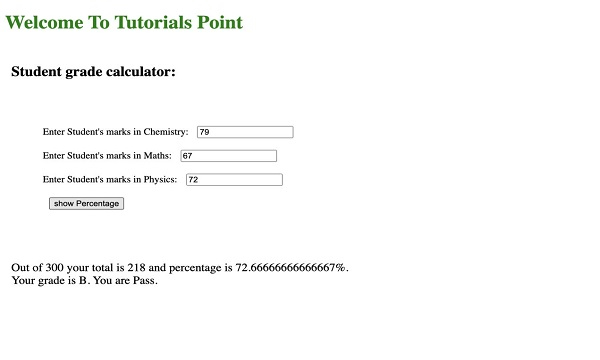# Creating a Simple Calculator using HTML, CSS, and JavaScript

A Student grade calculator is used for taking the grades input for all subjects and then calculating the percentage based upon the marks of the students. This calculator returns a fairly reliable indicator of student results.

The simple formula for calculating grades is:

$\text{Percentage}=\frac{\text{Marks Scored}}{\text{Total Marks}}\times 100$

We are going to take the inputs using HTML, once the inputs are entered we will call the JS function to calculate the average percentage of these numbers and will return the same to the user.

Steps for creating a calculator −

• We will be taking inputs from the user using the input tag.

• Once the inputs are taken, we will pass these inputs to the calculate function in JS.

• The calculate function will basically aggregate the result for this and return the result

• On getting the result, the HTML will display the result to the user.

## Example

In the below example, we are creating a simple grade calculator that will take grades inputs and find the aggregated grade percentage for each student.

index.html

<!DOCTYPE html>
<html>
<body>
<!-- main html -->
<h1 style="color: green">
Welcome To Tutorials Point
</h1>
<div class="container">
<div class="screen-body-item">
<div class="app">
<div class="form-group">
<!-- option for taking the input -->
Enter Student's marks in Chemistry:
<input
type="text"
class="form-control"
placeholder="CHEMISTRY"
id="chemistry" />
</div>
<div class="form-group">
Enter Student's marks in Maths:
<input
type="text"
class="form-control"
placeholder="MATHS"
id="maths" />
</div>
<div class="form-group">
Enter Student's marks in Physics:
<input
type="text"
class="form-control"
placeholder="PHYSICS"
id="phy" />
</div>
<div>
<input
type="button"
value="show Percentage"
class="form-button"
onclick="calculate()" />
</div>
</div>
</div>
<!-- for showing the result-->
<div class="form-group showdata">
<p id="showdata"></p>
</div>
</div>
<script src="script.js"></script>
</body>
</html>

styles.css

.container {
flex: 0 1 700px;
margin: auto;
}
.screen-body-item {
flex: 1;
}
input {
m   argin: 10px 10px 10px;
}
.showdata {
color: black;
font-size: 1.2rem;
}
#form-group {
}

script.js

// Function for calculating grades
const calculate = () => {
// Getting input from user into height variable.
let chemistry = document.querySelector("#chemistry").value;
let maths = document.querySelector("#maths").value;
let phy = document.querySelector("#phy").value;

// Input is string so typecasting is necessary. */
parseFloat(chemistry) +
parseFloat(maths) +
parseFloat(phy);

// Checking the condition for the providing the
// grade to student based on percentage
let percentage = (totalgrades / 300) * 100;
if (percentage <= 100 && percentage >= 80) {
} else if (percentage <= 79 && percentage >= 60) {
} else if (percentage <= 59 && percentage >= 40) {
} else {
}
// Checking the values are empty if empty than
if (chemistry == ""|| maths == "" || phy == "") { document.querySelector("#showdata").innerHTML = "Please enter all the fields";
} else {
// Checking the condition for the fail and pass
if (percentage >= 39.5) {
document.querySelector(
"#showdata"
).innerHTML =
Out of 300 your total is ${totalgrades} and percentage is${percentage}%. <br>
Your grade is ${grades}. You are Pass. ; } else { document.querySelector( "#showdata" ).innerHTML =  Out of 300 your total is${totalgrades}
and percentage is ${percentage}%. <br> Your grade is${grades}. You are Fail. ;
}
}
};

## OutputOn entering the marks and clicking on “show percentage” −We have merged the HTML, CSS, and JS to help you check the output of this program here itself.

Click the following link to see a Live Demo of this program: